Next: Voltage Divider Up: Kirchoff's Laws Previous: Kirchoff's Laws

## Series and Parallel Combinations of Resistors

Circuit elements are connected in series when a common current passes through each element. The equivalent resistance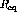of a combination of resistors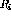connected in series is given by summing the voltage drops across each resistor.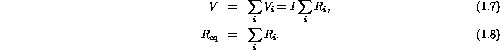If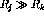, where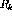are all the other resistors than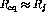; the largest resistor wins.

Circuit elements are connected in parallel when a common voltage is applied across each element. The equivalent resistanceof a combination of resistorsconnected in parallel is given by summing the current through each resistorIf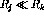, whereare all the other resistors than; the smallest resistor wins.

The following ``divider'' circuits are useful combinations of resistors. Believe it or not, they are a super useful concept that will often be used in one form or another; learn it.

Doug Gingrich
Tue Jul 13 16:55:15 EDT 1999